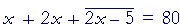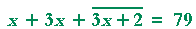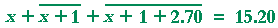S k i l l
i n
A L G E B R A

10

# WORD PROBLEMS

Examples

WORD PROBLEMS require practice in translating verbal language into algebraic language. See Lesson 1, Problem 8.  Yet, word problems fall into distinct types. Below are some examples.

Example 1.   ax ± b = c.  All problems like the following lead eventually to an equation in that simple form.

Jane spent \$42 for shoes.  This was \$14 less than twice what she spent for a blouse.  How much was the blouse?

Solution.   Every word problem has an unknown number. In this problem, it is the price of the blouse.  Always let x represent the unknown number.  That is, let x answer the question.

Let x, then, be how much she spent for the blouse.  The problem states that "This" -- that is, \$42 -- was \$14 less  than two times x.

Here is the equation:

 2x − 14 = 42. 2x = 42 + 14   (Lesson 9) = 56. x = 56 2 = 28.

The blouse cost \$28.

Example 2.   There are b boys in the class.  This is three more than four times the number of girls.  How many girls are in the class?

Solution.   Again, let x represent the unknown number that you are asked to find:  Let x be the number of girls.

(Although b is not known, it is not what you are asked to find.)

The problem states that "This" -- b -- is three more than four times x:

 4x + 3 = b. Therefore, 4x = b − 3 x = b − 3   4 .

The solution here is not a number, because it will depend on the value of b.  This is a type of "literal" equation, which is very common in algebra.

Example 3.  The whole is equal to the sum of the parts.

The sum of two numbers is 84, and one of them is 12 more than the other.  What are the two numbers?

Solution.  In this problem, we are asked to find two numbers. Therefore, we must let x be one of them.  Let x, then, be the first number.

We are told that the other number is 12 more, x + 12.

The problem states that their sum is 84:= 84

The line over x + 12 is a grouping symbol called a vinculum.  It saves us writing parentheses.

We have:

 2x = 84 − 12 = 72. x = 72 2 = 36.

This is the first number.  Therefore the other number is

x + 12 = 36 + 12 = 48.

The sum of 36 + 48 is 84.

Example 4.   The sum of two consecutive numbers is 37.  What are they?

Solution.   Two consecutive numbers are like 8 and 9, or 51 and 52.

Let x, then, be the first number.  Then the number after it is x + 1.

The problem states that their sum is 37:= 37

 2x = 37 − 1 = 36. x = 36 2 = 18.

The two numbers are 18 and 19.

Example 5.  One number is 10 more than another.  The sum of twice the smaller plus three times the larger, is 55.  What are the two numbers?

Solution.  Let x be the smaller number.

Then the larger number is 10 more:  x + 10.

The problem states:

 2x + 3(x + 10) = 55. That implies 2x + 3x + 30 = 55.  Lesson 14. 5x = 55 − 30 = 25. x = 5.

That's the smaller number.  The larger number is 10 more:  15.

Example 6.   Divide \$80 among three people so that the second will have twice as much as the first, and the third will have \$5 less than the second.

Solution.   Again, we are asked to find more than one number.  We must begin by letting x be how much the first person gets.

Then the second gets twice as much, 2x.

And the third gets \$5 less than that, 2x − 5.

Their sum is \$80:5x = 80 + 5 x = 85 5 = 17.

This is how much the first person gets.  Therefore the second gets

 2x = 34. And the third gets 2x − 5 = 29.

The sum of 17, 34, and 29 is in fact 80.

Example 7.  Odd numbers.   The sum of two consecutive odd numbers is 52.  What are the two odd numbers?

Solution.   First, an even number is a multiple of 2:  2, 4, 6, 8, and so on. It is conventional in algebra to represent an even number as 2n, where, by calling the variable 'n,' it is understood that n will take whole number values:  n = 0, 1, 2, 3, 4, and so on.

An odd number is 1 more (or 1 less) than an even number. And so we represent an odd number as 2n + 1.

Let 2n + 1, then, be the first odd number. Then the next one will be 2 more -- it will be 2n + 3.  The problem states that their sum is 52:

 2n + 1  +  2n + 3 = 52

We will now solve that equation for n, and then replace the solution in 2n + 1 to find the first odd number.  We have:

 4n + 4 = 52 4n = 48 n = 12.

Therefore the first odd number is  2· 12 + 1 = 25.  And so the next one is 27.  Their sum is 52.

Problems

Problem 1.   Julie has \$50, which is eight dollars more than twice what John has.  How much has John?  (Compare Example 1.)

First, what will you let x represent?

To see the answer, pass your mouse over the colored area.
To cover the answer again, click "Refresh" ("Reload").
Do the problem yourself first!

The unknown number -- which is how much that John has.

What is the equation?

2x + 8 = 50.

Here is the solution:

x = \$21

Problem 2.   Carlotta spent \$35 at the market.  This was seven dollars less than three times what she spent at the bookstore; how much did she spend there?

Here is the equation.

3x − 7 = 35

Here is the solution:

x = \$14

Problem 3.   There are b black marbles.  This is four more than twice the number of red marbles.  How many red marbles are there?  (Compare Example 2.)

Here is the equation.

2x + 4 = b

Here is the solution:

 x = b − 4   2

Problem 4.    Janet spent \$100 on books.  This was k dollars less than five times what she spent on lunch.  How much did she spend on lunch?

Here is the equation.

5xk = 100

Here is the solution:

 x = 100 + k     5

Problem 5.  The whole is equal to the sum of the parts.

The sum of two numbers is 99, and one of them is 17 more than the other.  What are the two numbers?  (Compare Example 3.)

Here is the equation.Here is the solution:

 x = 41 x + 17 = 58

Problem 6.   A class of 50 students is divided into two groups; one group has eight less than the other; how many are in each group?

Here is the equation.Here is the solution:

 x = 29 x − 8 = 21

Problem 7.   The sum of two numbers is 72, and one of them is five times the other; what are the two numbers?

Here is the equation.

x + 5x = 72.

Here is the solution:

x = 12.   5x = 60.

Problem 8.   The sum of three consecutive numbers is 87; what are they?  (Compare Example 4.)

Here is the equation.Here is the solution:

28, 29, 30.

Problem 9.   A group of 266 persons consists of men, women, and children.  There are four times as many men as children, and twice as many women as children.  How many of each are there?

(What will you let x equal -- the number of men, women, or children?)

 Let x = The number of children.  Then 4x = The number of men. And 2x = The number of women. Here is the equation:

x + 4x + 2x = 266

Here is the solution:

x = 38.  4x = 152.  2x = 76.

Problem 10.   Divide \$79 among three people so that the second will have three times more than the first, and the third will have two dollars more than the second.   (Compare Example 6.)

Here is the equation.Here is the solution:

\$11,  \$33,  \$35.

Problem 11.   Divide \$15.20 among three people so that the second will have one dollar more than the first, and the third will have \$2.70 more than the second.

Here is the equation.Here is the solution:

\$3.50,  \$4.50,  \$7.20.

Problem 12.   Two consecutive odd numbers are such that three times the first is 5 more than twice the second.  What are those two odd numbers?

(See Example 7, where we represent an odd number as 2n + 1.)

Solution. Let the first odd number be 2n + 1.

Then the next one is 2n + 3 -- because it will be 2 more.

The problem states, that is, the equation is:

 3(2n + 1) = 2(2n + 3) + 5. That implies: 6n + 3 = 4n + 6 + 5. 2n = 8. n = 4.

Therefore the first odd number is 2· 4 + 1 = 9.  The next one is 11.

And that is the true solution, because according to the problem:

3· 9 = 2· 11 + 5.Next Lesson:  Inequalities

Please make a donation to keep TheMathPage online.
Even \$1 will help.